# 一步步教你用 WebVR 实现虚拟现实游戏

level

0收藏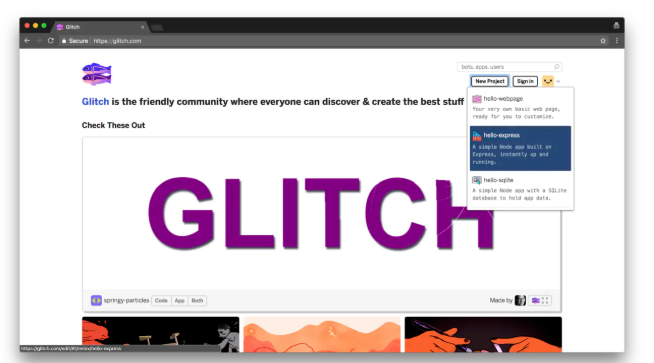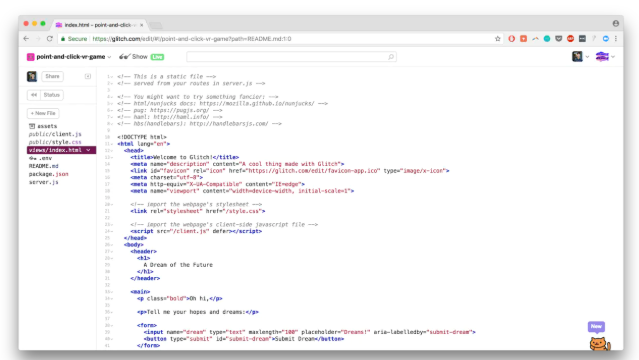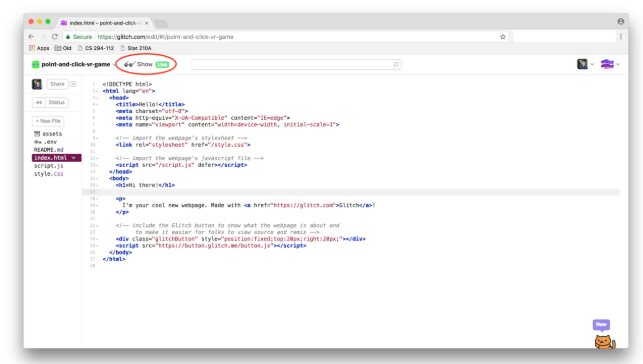``````<!DOCTYPE html>
<html>
<script src="https://aframe.io/releases/0.7.0/aframe.min.js"></script>
<body>
<a-scene>

<!-- blue sky -->
<a-sky color="#a3d0ed"></a-sky>

<!-- camera with wasd and panning controls -->
<a-entity camera look-controls wasd-controls position="0 0.5 2" rotation="0 0 0"></a-entity>

<!-- brown ground -->

<!-- start code here -->
<!-- end code here -->
</a-scene>
</body>
</html>``````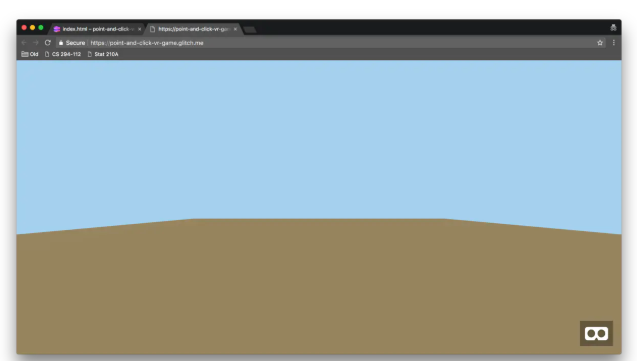Aframe 通过定义基元来简化这个概念，例如 <a-box>，<a-sphere>，<a-cylinder> 以及许多其他基本原理来简化几何体及其材料。首先定义一个绿色球体。在代码的第19行，也就是 <!-- start code here -->之后添加以下内容。

<!-- start code here -->
<a-sphere color="green" radius="0.5"></a-sphere>  <!-- new line -->
<!-- end code here -->

<!-- start code here -->
<a-sphere color="green" radius="0.5" position="0 1 0"></a-sphere> <!-- edited line -->
<!-- end code here -->

<a-sphere color="green" radius="0.5" position="0 1 0">
<a-cylinder color="#84651e" position="0 -0.9 0" radius="0.05"></a-cylinder> <!-- new line -->
</a-sphere>

<a-sphere color="green" radius="0.5" position="0 0.75 0">
<a-cylinder color="#84651e" position="0 -0.9 0" radius="0.05"></a-cylinder>
<a-sphere color="green" radius="0.35" position="0 0.5 0"></a-sphere> <!-- new line -->
<a-sphere color="green" radius="0.2" position="0 0.8 0"></a-sphere> <!-- new line -->
</a-sphere>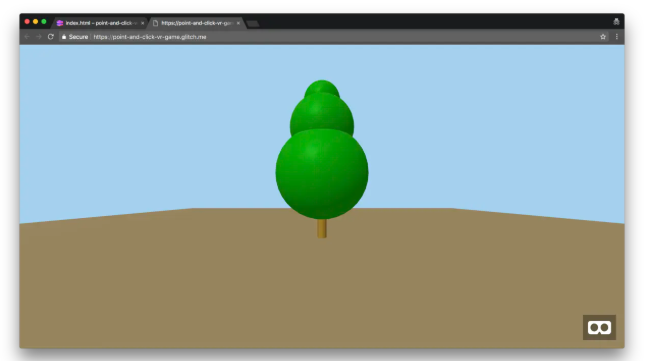<a-entity camera look-controls wasd-controls position="0 0.5 2" rotation="0 0 0">
<a-entity cursor="fuse: true; fuseTimeout: 250"
position="0 0 -1"
scale="0.5 0.5 0.5"
raycaster="far: 20; interval: 1000; objects: .clickable">
</a-entity>
</a-entity>

<a-animation begin="fusing" easing="ease-in" attribute="scale"
fill="backwards" from="1 1 1" to="0.2 0.2 0.2" dur="250"></a-animation>

<a-sphere color="green" radius="0.5" position="2 0.75 0" class="clickable">

<a-animation begin="click" attribute="position" from="2 0.75 0" to="2.2 0.75 0" fill="both" direction="alternate" repeat="1"></a-animation>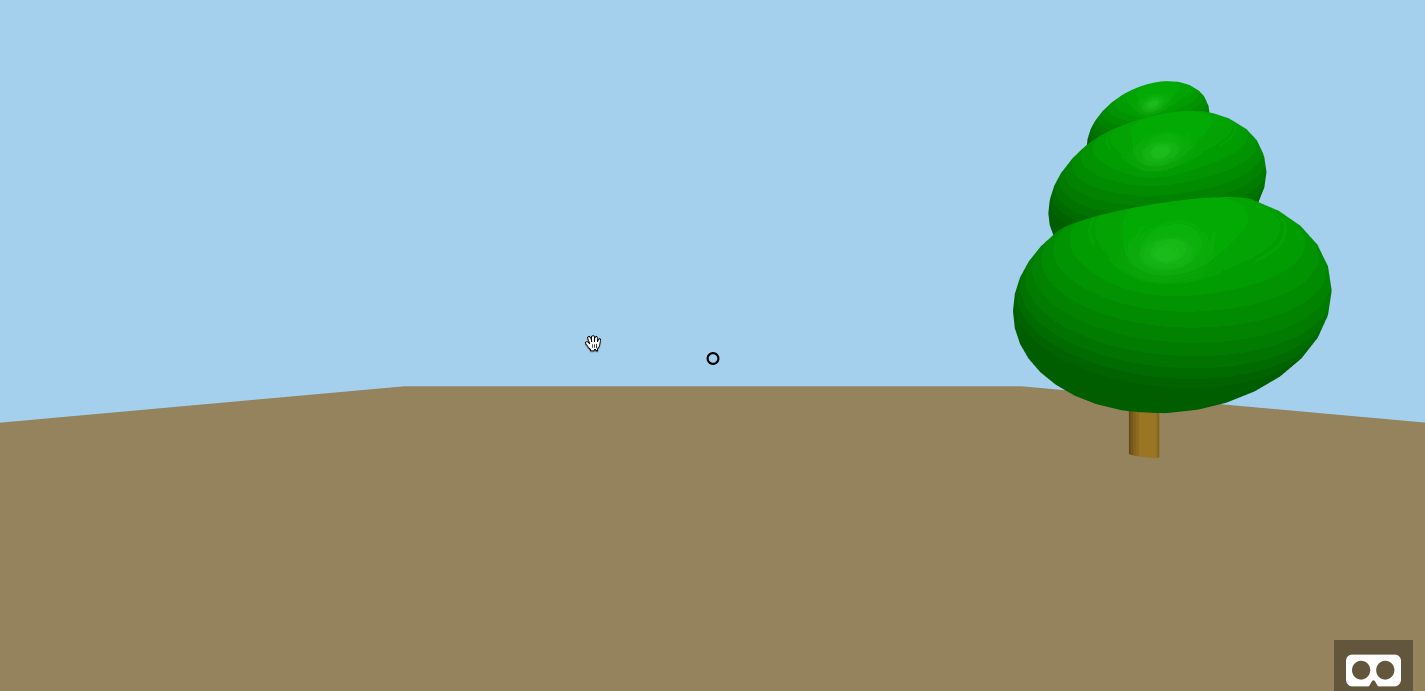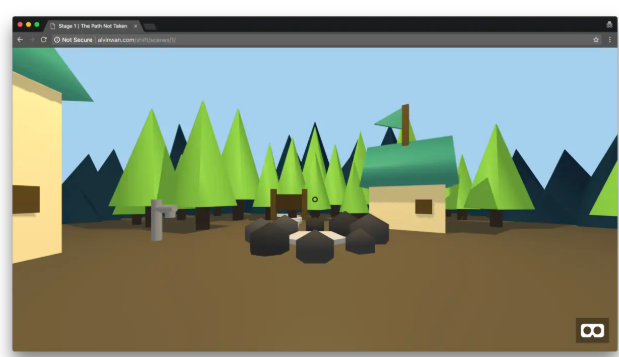帖子
视频
声望
粉丝
社区精华内容